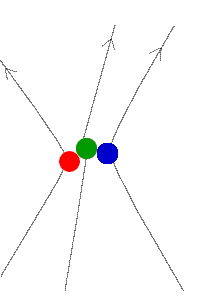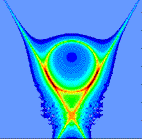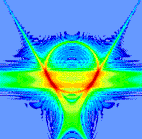The Ilya Prigogine Center for Studies in Statistical Mechanics and Complex Systems Back the Center Homepage | About the Author | Previous | NextPage 2 The three-paneled diagram at right is an example of how the scientists at the Center have studied the three-body scattering system. Each diagram represents ``mathematical portrait" of the state of the system at a certain time in its evolution.Each diagram is a mathematical plot in which values on both the horizontal and vertical axis are calculated from the relative speedsof the particles at a certain instant of time. The top diagram represents the system after the collision, and the bottom diagram represents the system during the collision.After the Collision The colors in the plot represent the probability (at that time) of measuring the system with a a certain value of relative speeds. One reason that probabilities must be used here because the system studied here is quantum mechanical, rather than classical.During the Collision Next

Text and Graphics (c) 1999 by Matthew Trump. Original three-panel diagrams by T. Miyasaka